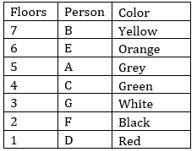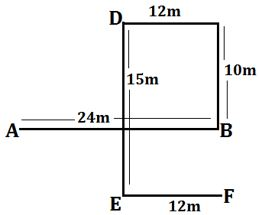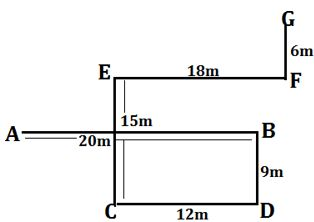Latest Banking jobs   »

# Reasoning Ability Quiz For IBPS Clerk/PO Prelims 2022- 27th August

Directions (1-5): Study the following information carefully and answer the following questions.
Seven people live on seven different floors from top to bottom such that ground floor is numbered as 1 and so on up to top floor is numbered as 7. They all like different colours i.e. Yellow, Orange, Grey, Green, White, Black, and Red.
C lives on an even numbered floor and likes Green. There are two floors between the floor on which C and D lives. G lives just above the floor on which F lives who likes Black. There are two floors between the floor on which F and A lives. A lives on one of the above floors on which floor G lives. B lives on one of the above floors on which E lives. E lives neither on first nor fifth number floor. There are two people live between the one who likes Yellow and C. Only two people live between the one who likes Red and the one who likes Green. B doesn’t like Red. The person who likes Orange lives immediate above than the person who likes Grey.

Q1. How many people live between D and E?
(a) One
(b) Three
(c) Four
(d) Two
(e) None of these

Q2. What is the correct combination for the person who lives on floor number Seven?
(a) D, Green
(b) C, Grey
(c) A, Orange
(d) B, Yellow
(e) F, Black

Q3. How many persons live between F and the one who likes Grey?
(a) One
(b) Two
(c) Five
(d) Three
(e) None of these

Q4. Who likes White?
(a) G
(b) H
(c) A
(d) E
(e) C

Q5. Four of the following five are alike in a certain way based on the given arrangement and thus form a group. Which is the one that does not like that group?
(a) F
(b) D
(c) G
(d) B
(e) A

Directions (6-10): Study the following arrangement carefully and answer the questions given below:

G C @ 5 U © T Z 7 3 = F 6 7 M 2 & B % 4 H £ 9 \$ A W K π 8 E L

Q6. If all vowels in the above arrangement are dropped, then which of the following will be the 10th from the right end?
(a) B
(b) H
(c) &
(d) K
(e) None of these

Q7. How many such consonants are there in the above arrangement each of which is immediately preceded by symbol but not immediately followed by a vowel?
(a) None
(b) One
(c) Two
(d) Three
(e) More than three

Q8. Which of the following is the 10th to the left of the 18th element from the left end of the above arrangement?
(a) %
(b) B
(c) T
(d) Z

Q9. How many such vowels are there in the above arrangement each of which is immediately followed by symbol but not immediately preceded by a number?
(a) None
(b) One
(c) Two
(d) Three
(e) None of these

Q10. If all symbols in the above arrangement are dropped, then which of the following will be the 15th from the left end?
(a) H
(b) A
(c) 4
(d) M
(e) None of these

Direction (11-12): Study the following information carefully and answer the given questions:
A person starts walking from point A to the east direction and walks 24m to reach at point B. From point B he takes two consecutive left turns and walks 10m and 12m respectively and reach at point D. From point D he walks in south direction and walks 15m to reach at point E, then finally turn his left and walks 12m to reach final point F.

Q11. What is the direction of his starting point with respect to point D?
(a) North-west
(b) South-West
(c) North-east
(d) South
(e) None of these

Q12. If point O is the midpoint of point A and B, then what is the shortest distance between point O to his final position?
(a) 15m
(b) 12m
(c) 10m
(d) 13m
(e) None of these

Direction (13-15): Study the following information carefully and answer the given questions:
Point A is 20m west of point B, which is 9m north of point D. Point F is 6m south of point G. Point E is 15m north of point C. Point F is 18m east of point E. Point C is 12m west of point D.

Q13. What is the direction of point G with respect to point D?
(a) South
(b)North-east
(c)South-west
(d)East
(e) None of these

Q14. What is the shortest distance between point E to point A?
(a)12m
(b)18m
(c) 25m
(d) 10m
(e) None of these

Q15. What is the direction of point A with respect to point C?
(a) North-West
(b)South-East
(c)North-East
(d) West
(e) None of these

Solutions

Solutions (1-5):
Sol.S1. Ans. (c)
S2. Ans. (d)
S3. Ans. (b)
S4. Ans. (a)
S5. Ans. (a)

S6. Ans. (e)
S7. Ans. (d)
S8. Ans. (d)
S9. Ans. (a)
S10. Ans. (c)

Solutions (11-12):
Sol.S11. Ans. (b)
S12. Ans. (d)

Solutions (13-15):
Sol.S13. Ans. (b)
S14. Ans. (d)
S15. Ans. (a)#### Congratulations!Download Hindu Review of October 2021: Free PDF